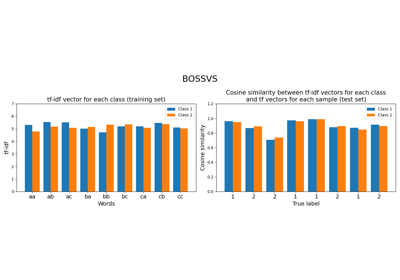# `pyts.classification`.BOSSVS¶

class `pyts.classification.``BOSSVS`(word_size=4, n_bins=4, window_size=10, window_step=1, anova=False, drop_sum=False, norm_mean=False, norm_std=False, strategy='quantile', alphabet=None, numerosity_reduction=True, use_idf=True, smooth_idf=False, sublinear_tf=True)[source]

Bag-of-SFA Symbols in Vector Space.

Each time series is transformed into an histogram using the Bag-of-SFA Symbols (BOSS) algorithm. Then, for each class, the histograms are added up and a tf-idf vector is computed. The predicted class for a new sample is the class giving the highest cosine similarity between its tf vector and the tf-idf vectors of each class.

Parameters: word_size : int (default = 4) Size of each word. n_bins : int (default = 4) The number of bins to produce. It must be between 2 and 26. window_size : int or float (default = 10) Size of the sliding window. If float, it represents the percentage of the size of each time series and must be between 0 and 1. The window size will be computed as `ceil(window_size * n_timestamps)`. window_step : int or float (default = 1) Step of the sliding window. If float, it represents the percentage of the size of each time series and must be between 0 and 1. The window size will be computed as `ceil(window_step * n_timestamps)`. anova : bool (default = False) If True, the Fourier coefficient selection is done via a one-way ANOVA test. If False, the first Fourier coefficients are selected. drop_sum : bool (default = False) If True, the first Fourier coefficient (i.e. the sum of the subseries) is dropped. Otherwise, it is kept. norm_mean : bool (default = False) If True, center each subseries before scaling. norm_std : bool (default = False) If True, scale each subseries to unit variance. strategy : str (default = ‘quantile’) Strategy used to define the widths of the bins: ‘uniform’: All bins in each sample have identical widths ‘quantile’: All bins in each sample have the same number of points ‘normal’: Bin edges are quantiles from a standard normal distribution ‘entropy’: Bin edges are computed using information gain alphabet : None, ‘ordinal’ or array-like, shape = (n_bins,) Alphabet to use. If None, the first n_bins letters of the Latin alphabet are used. numerosity_reduction : bool (default = True) If True, delete sample-wise all but one occurence of back to back identical occurences of the same words. use_idf : bool (default = True) Enable inverse-document-frequency reweighting. smooth_idf : bool (default = False) Smooth idf weights by adding one to document frequencies, as if an extra document was seen containing every term in the collection exactly once. Prevents zero divisions. sublinear_tf : bool (default = True) Apply sublinear tf scaling, i.e. replace tf with 1 + log(tf).

References

  P. Schäfer, “Scalable Time Series Classification”. Data Mining and Knowledge Discovery, 30(5), 1273-1298 (2016).

Examples

```>>> from pyts.classification import BOSSVS
>>> X_train, X_test, y_train, y_test = load_gunpoint(return_X_y=True)
>>> clf = BOSSVS(window_size=28)
>>> clf.fit(X_train, y_train)
BOSSVS(...)
>>> clf.score(X_test, y_test)
0.98
```
Attributes: classes_ : array, shape = (n_classes,) An array of class labels known to the classifier. idf_ : array, shape = (n_features,) , or None The learned idf vector (global term weights) when `use_idf=True`, None otherwise. tfidf_ : array, shape = (n_classes, n_words) Term-document matrix. vocabulary_ : dict A mapping of feature indices to terms.

Methods

 `__init__`([word_size, n_bins, window_size, …]) Initialize self. `decision_function`(X) Evaluate the cosine similarity between document-term matrix and X. `fit`(X, y) Compute the document-term matrix. `get_params`([deep]) Get parameters for this estimator. `predict`(X) Predict the class labels for the provided data. `score`(X, y[, sample_weight]) Return the mean accuracy on the given test data and labels. `set_params`(**params) Set the parameters of this estimator.
`__init__`(word_size=4, n_bins=4, window_size=10, window_step=1, anova=False, drop_sum=False, norm_mean=False, norm_std=False, strategy='quantile', alphabet=None, numerosity_reduction=True, use_idf=True, smooth_idf=False, sublinear_tf=True)[source]

Initialize self. See help(type(self)) for accurate signature.

`decision_function`(X)[source]

Evaluate the cosine similarity between document-term matrix and X.

Parameters: X : array-like, shape (n_samples, n_timestamps) Test samples. X : array, shape (n_samples, n_classes) Cosine similarity between the document-term matrix and X.
`fit`(X, y)[source]

Compute the document-term matrix.

Parameters: X : array-like, shape = (n_samples, n_timestamps) Training vector. y : array-like, shape = (n_samples,) Class labels for each data sample. self : object
`get_params`(deep=True)

Get parameters for this estimator.

Parameters: deep : bool, default=True If True, will return the parameters for this estimator and contained subobjects that are estimators. params : dict Parameter names mapped to their values.
`predict`(X)[source]

Predict the class labels for the provided data.

Parameters: X : array-like, shape = (n_samples, n_timestamps) Test samples. y_pred : array, shape = (n_samples,) Class labels for each data sample.
`score`(X, y, sample_weight=None)

Return the mean accuracy on the given test data and labels.

Parameters: X : array-like, shape = (n_samples, n_timestamps) Univariate time series. y : array-like, shape = (n_samples,) True labels for X. sample_weight : None or array-like, shape = (n_samples,) (default = None) Sample weights. score : float Mean accuracy of `self.predict(X)` with regards to y.
`set_params`(**params)

Set the parameters of this estimator.

The method works on simple estimators as well as on nested objects (such as `Pipeline`). The latter have parameters of the form `<component>__<parameter>` so that it’s possible to update each component of a nested object.

Parameters: **params : dict Estimator parameters. self : estimator instance Estimator instance.

## Examples using `pyts.classification.BOSSVS`¶Bag-of-SFA Symbols in Vector Space (BOSSVS)